Next: Growing Cell Structures Up: Soft Competitive Learning with Previous: Soft Competitive Learning with

# Self-organizing Feature Map

This model stems from Kohonen (1982) and builds upon earlier work of Willshaw and von der Malsburg (1976). The model is similar to the (much later developed) neural gas model (see 5.1) since a decaying neighborhood range and adaptation strength are used. An important difference, however, is the topology which is constrained to be a two-dimensional grid (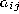) and does not change during self-organization.

The distance on this grid is used to determine how strongly a unitis adapted when the unit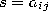is the winner. The distance measure is the-norm (a.k.a. ``Manhattan distance''):Ritter et al. (1991) propose to use the following function to define the relative strength of adaptation for an arbitrary unit r in the network (given that s is the winner):Thereby, the standard deviationof the Gaussian is varied according tofor a suitable initial value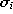and a final value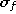. The complete self-organizing feature map algorithm is the following:

1. Initialize the setto containunits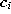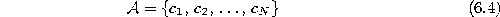with reference vectorschosen randomly according to.

Initialize the connection setto form a rectangulargrid.

Initialize the time parameter t:2. Generate at random an input signalaccording to.
3. Determine the winner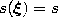:4. Adapt each unit r according towherebyand5. Increase the time parameter t:6. Ifcontinue with step 2.

Figure 6.1 shows some stages of a simulation for a simple ring-shaped data distribution. Figure 6.2 displays the final results after 40000 adaptation steps for three other distribution. The parameters wereand.Figure 6.1:   Self-organizing feature map simulation sequence for a ring-shaped uniform probability distribution. a) Initial state. b-f) Intermediate states. g) Final state. h) Voronoi tessellation corresponding to the final state. Large adaptation rates in the beginning as well as a large neighborhood range cause strong initial adaptations which decrease towards the end.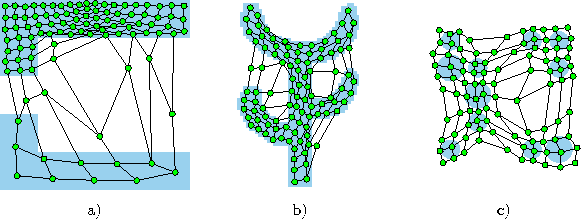Figure:   Self-organizing feature map simulation results after 40000 input signals for three different probability distributions (described in the caption of figure 4.4).Next: Growing Cell Structures Up: Soft Competitive Learning with Previous: Soft Competitive Learning with

Bernd Fritzke
Sat Apr 5 18:17:58 MET DST 1997